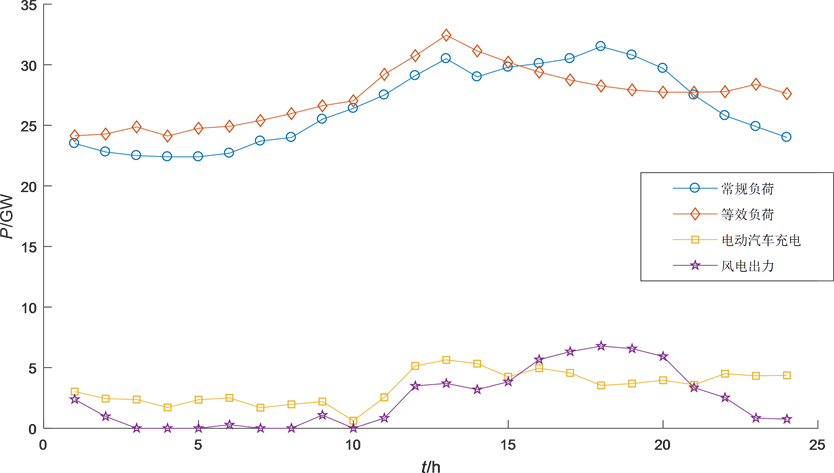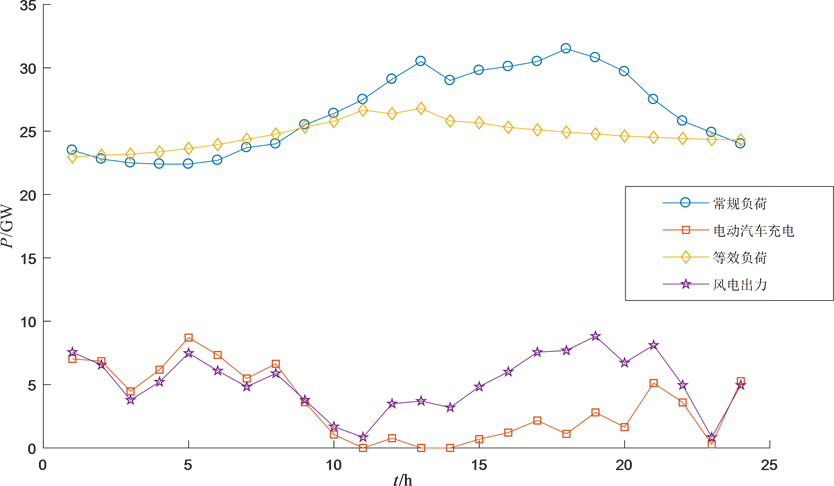# 考虑电动汽车生命周期碳排放的风电协同调度策略Optimal Scheduling Strategy Considering the Life Cycle Carbon Emissions for Electric Vehicles Cooperating with Wind Power

DOI: 10.12677/SG.2018.83029, PDF, HTML, XML, 下载: 455  浏览: 1,554  国家科技经费支持

Abstract: Under the background of low-carbon economy, this paper comprehensively considers environmental and economic benefits, and proposes a coordinated dispatch model for electric vehicles and wind power with the goal of the lowest carbon emissions, the lowest cost of thermal power, and the lowest peak-to-valley equivalent load. In terms of carbon emissions, this paper has calculated the carbon emissions of electric vehicles throughout their life cycle. In the cost section, the carbon trading mechanism was added to fully consider the economic value of carbon emission reduction. This paper uses the multi-objective particle swarm optimization to solve the problems and compare it with the scenarios of disordered charging of fuel vehicles and electric vehicles to illustrate the feasibility and effectiveness.

1. 引言

2. 电动汽车全生命周期碳排放水平

${E}_{cl}=\underset{i}{\sum }{E}_{i}×{p}_{i}$ (1)

${E}_{zz}=\frac{{E}_{all}}{M}$ (2)

$TC{E}_{l}=\rho ×\left({E}_{cl}+{E}_{zz}\right)$ (3)

${I}_{ev}=\frac{{I}_{sum}}{{S}_{sum}\cdot \left(W/100\right)\cdot {\eta }_{c}}$ (4)

3. 电动汽车充电负荷建模与风电出力建模

${f}_{t}=\left\{\begin{array}{l}\frac{1}{{\sigma }_{1}\sqrt{2\text{π}}}\mathrm{exp}\left[-\frac{{\left(t-{\mu }_{1}\right)}^{2}}{2{\sigma }_{1}{}^{2}}\right]\text{}\text{\hspace{0.17em}}\text{\hspace{0.17em}}\left({\mu }_{1}-12\right) (5)

$f\left({S}_{0}\right)\frac{1}{{\sigma }_{0}\sqrt{2\text{π}}}\mathrm{exp}\left[-\frac{{\left(s-{\mu }_{0}\right)}^{2}}{2{\sigma }_{0}{}^{2}}\right]$ (6)

$f\left({S}_{t}\right)\frac{1}{{\sigma }_{t}\sqrt{2\text{π}}}\mathrm{exp}\left[-\frac{{\left(s-{\mu }_{t}\right)}^{2}}{2{\sigma }_{t}{}^{2}}\right]$ (7)

${f}_{V}\left(v\right)=\frac{k}{c}{\left(\frac{v}{c}\right)}^{k-1}\mathrm{exp}\left[-{\left(\frac{v}{c}\right)}^{k}\right]$ (8)

${p}^{w}=\left\{\begin{array}{l}0\text{}v<{v}_{in}或v>{v}_{out}\\ {p}_{r}^{w}\frac{v-{v}_{in}}{{v}_{r}-{v}_{in}}\text{}{v}_{in}\le v<{v}_{r}\\ {p}_{r}^{w}\text{}{v}_{r}\le v\le {v}_{out}\end{array}$ (9)

4. 电动汽车-风电协同调度建模

4.1. 目标函数

$\mathrm{min}{E}_{h}\underset{t=1}{\overset{n}{\sum }}{P}_{h,t}+{I}_{ev}\underset{t=1}{\overset{n}{\sum }}{P}_{ev,t}$ (10)

$\mathrm{min}\underset{t=1}{\overset{N}{\sum }}{C}_{t}^{gas}+{C}_{t}^{c}$ (11)

${C}_{t}^{gas}=\underset{i=1}{\overset{N}{\sum }}\left({a}_{i}+{b}_{i}{P}_{i,t}+{c}_{i}{P}_{i,t}\right)$ (12)

${C}_{t}^{c}=K\left({P}_{c,t}-{M}_{t}\right)$ (13)

${M}_{t}=\underset{i=1}{\overset{N}{\sum }}\epsilon {P}_{i,t}$ (14)

$\mathrm{min}\underset{i=1}{\overset{T-M+1}{\sum }}\underset{t=i}{\overset{i+M-1}{\sum }}{\left({P}_{l,t}-{P}_{w,t}+{P}_{ev,t}-{P}_{av,i}\right)}^{2}$ (15)

${P}_{av,i}=\frac{1}{M}\underset{t=i}{\overset{i+M-1}{\sum }}\left({P}_{l,t}-{P}_{w,t}+{P}_{ev,t}\right)$ (16)

4.2. 约束条件

1) 系统约束

$\underset{i=1}{\overset{N}{\sum }}{P}_{i,t}+{P}_{w,t}={P}_{l,t}+{P}_{ev,t}$ (17)

2) 电动汽车充电约束

$0\le {P}_{ev,t}\le {P}_{ch,t}$ (18)

${S}_{\mathrm{min}}\le {S}_{t}\le {S}_{\mathrm{max}}$ (19)

$\underset{t=1}{\overset{T}{\sum }}{P}_{ev,t}\ge {P}_{f}$ (20)

3) 火电机组出力约束

a) 出力约束

${P}_{i}^{\mathrm{min}}\le {P}_{i,t}\le {P}_{i}^{\mathrm{max}}$ (21)

b) 爬坡约束

$-{R}_{i}^{D}\Delta t\le {P}_{i,t}-{P}_{i,t-1}\le {R}_{i}^{U}\Delta t$ (22)

c) 风电出力约束

$0\le {P}_{w,t}\le {P}_{w,t}^{\mathrm{max}}$ (23)

4.3. 模型求解

1) 随机初始化种群，设定惯性权值。

2) 求解粒子的各目标函数值。

3) 按照适应度对粒子进行非支配排序。

4) 计算各粒子的拥挤距离。

5) 根据粒子的前沿等级和拥挤距离，使用锦标赛选择法选取种群的个体最优和全局最优。

6) 按照下式更新粒子：

$\begin{array}{c}{V}_{i,j}\left(t+1\right)=\omega {V}_{i,j}\left(t\right)+{c}_{1}×rand\left(\right)×\left[pbes{t}_{i,j}-{x}_{i,j}\left(t\right)\right]\\ \text{\hspace{0.17em}}\text{\hspace{0.17em}}+{c}_{2}×rand\left(\text{ }\right)×\left[gbes{t}_{i,j}-{x}_{i,j}\left(t\right)\right]\end{array}$ (24)

$\text{}{x}_{i,j}\left(t+1\right)={x}_{i,j}\left(t\right)+{V}_{i,j}\left(t+1\right)$ (25)

${V}_{i}$ 是粒子的速度，pbest和gbest是粒子的个体最佳和全局最佳位置，rand代表0~1之间的随机数，c1、c2是学习因子，ω代表惯性权值。

7) 重复2~6过程直至满足迭代条件。

${\omega }_{i}=\left\{\begin{array}{l}1\text{}{f}_{i}\le {f}_{i}^{\mathrm{min}}\\ \frac{{f}_{i}^{\mathrm{max}}-{f}_{i}}{{f}_{i}^{\mathrm{max}}-{f}_{i}^{\mathrm{min}}}\text{}{f}_{i}^{\mathrm{max}}>{f}_{i}>{f}_{i}^{\mathrm{min}}\\ 0\text{}{f}_{i}\ge {f}_{i}^{\mathrm{max}}\end{array}$ (26)

$\omega =\frac{1}{m}\underset{i=1}{\overset{m}{\sum }}{\omega }_{i}$ (27)

5. 算例分析Figure 1. 24-hour wind power output curveFigure 2. Free charge of electric carsFigure 3. Coordinated charge of electric carsTable 1. Comparison of free charge and co-chargeTable 2. Carbon emissions per mile of different wind power capacity

6. 结论

  于大洋, 宋曙光, 张波, 韩学山. 区域电网电动汽车充电与风电协同调度的分析[J]. 电力系统自动化, 2011, 35(14): 25-29.  Pavić, I., Capuder, T. and Holjevac, N. (2015) Role and Impact of Coordinated EV Charging on Flexibility in Low Carbon Power Systems. Electric Vehicle Conference, Florence, 17-19 December 2014, 1-8.  Yamashita, D. and Niimura, T. (2012) Opti-mal Strategy to Support the Development of Charging Infrastructure for Electric Vehicles towards Low Carbon Emissions. Power & Energy Society General Meeting, San Diego, CA, 22-26 July 2012, 1-7. https://doi.org/10.1109/PESGM.2012.6345451  于大洋, 黄海丽, 雷鸣, 等. 电动汽车充电与风电协同调度的碳减排效益分析[J]. 电力系统自动化, 2012, 36(10): 14-18.  侯建朝, 胡群丰, 谭忠富. 计及需求响应的风电-电动汽车协同调度多目标优化模型[J]. 电力自动化设备, 2016, 36(7): 22-27.  施泉生, 邸超, 孙佳佳, 张圣富, 李士动. 风电与电动汽车协同并网调度环境及经济模型[J]. 上海电力学报, 2017, 33(2): 113-118.  张静, 汤奕, 陈成. 考虑分时电价和系统峰谷差动态约束的电动汽车有序充电策略[J]. 电网与清洁能源, 2014, 30(5): 79-84.  魏大钧, 张承慧, 孙波. 基于分时电价的电动汽车充放电多目标优化调度[J]. 电网技术, 2014, 38(11): 2972-2977.  葛少云, 王龙, 刘洪, 等. 计及电动汽车入网的峰谷电价分时段优化模型研究[J]. 电网技术, 2013, 37(8): 2316-2321.  孙波, 孙佳佳, 董浩. 基于分时充电电价的电动汽车消纳风电的机组调度优化模型[J]. 可再生能源, 2017, 35(1): 110-118.  佟晶晶, 温俊强, 王丹, 等. 基于分时电价的电动汽车多目标优化充电策略[J]. 电力系统保护与控制, 2016, 44(1): 17-23.  刘志珍, 杨勇, 屈东明, 等. 基于分时电价的电动汽车群有序充电策略研究[J]. 电机与控制学报, 2017, 21(10): 1-7.  徐智威, 胡泽春, 宋永华, 等. 基于动态分时电价的电动汽车充电站有序充电策略[J]. 中国电机工程学报, 2014, 32(22): 3638-3646.  Skarvelis-Kazakos, S., Papadopoulos, P. and Grau, I. (2010) Carbon Optimized Virtual Power Plant with Electric Vehicles. Universities Power Engineering Conference, Cardiff, 31 August-3 September 2010, 1-6.  黄贵鸿, 雷霞, 杨毅. 考虑风电与用户满意度的电动汽车两层智能充放电策略[J]. 电工技术学报, 2015, 30(5): 85-97.  刘祚宇, 齐峰, 文福拴, 张西竹, 王蕾. 含电动汽车虚拟电厂参与碳交易时的经济与环境调度[J]. 电力建设, 2017, 38(9): 45-52.  潘湘源. 基于生命周期理论的纯电动汽车碳排放研究[D]: [硕士学位论文]. 上海: 同济大学, 2014.  许上宇. 含风光储的离网型电动汽车充电站容量配比研究[D]: [硕士学位论文]. 成都: 电子科技大学, 2016.  裴胜玉, 周永权. 基于Pareto最优解集的多目标粒子群优化算法[J]. 计算机工程与科学, 2010, 32(11): 85-88.  董安有. 我国解决风电弃风的组合途径及优化模型研究[D]: [博士学位论文]. 北京: 华北电力大学.